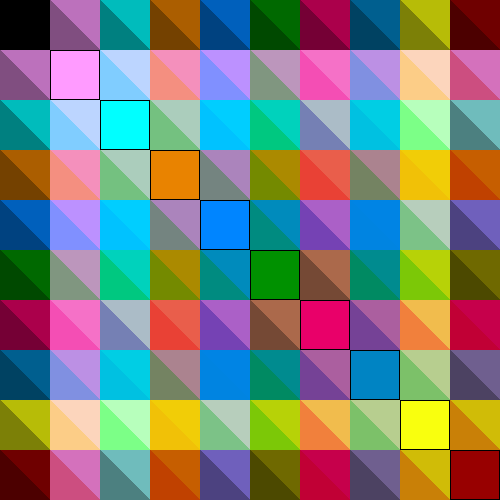dummy-link

20

6

18

16

# ColorVectorSpace

This package is an add-on to ColorTypes, and provides fast mathematical operations for objects with types such as RGB and Gray. Specifically, with this package both grayscale and RGB colors are treated as if they are points in a normed vector space.

## Introduction

Colorspaces such as RGB, unlike XYZ, are technically non-linear; perhaps the most "colorimetrically correct" approach when averaging two RGBs is to first convert each to XYZ, average them, and then convert back to RGB. Nor is there a clear definition of computing the sum of two colors. As a consequence, Julia's base color package, ColorTypes, does not support mathematical operations on colors.

However, particularly in image processing it is common to ignore this concern, and for the sake of performance treat an RGB as if it were a 3-vector. The role of this package is to extend ColorTypes to support such mathematical operations. Specifically, it defines + and multiplication by a scalar (and by extension, - and division by a scalar) for grayscale and AbstractRGB colors. These are the requirements of a vector space.

If you're curious about how much the "colorimetrically correct" and "vector space" views differ, the following diagram might help. The first 10 distinguishable_colors were generated, and all pairs were averaged. Each box represents the average of the pair of diagonal elements intersected by tracing vertically and horizontally; within each box, the upper diagonal is the "colorimetrically-correct" version, while the lower diagonal represents the "RGB vector space" version.This package also defines norm(c) for RGB and grayscale colors. This makes these color spaces normed vector spaces. Note that norm has been designed to satisfy equivalence of grayscale and RGB representations: if x is a scalar, then norm(x) == norm(Gray(x)) == norm(RGB(x, x, x)). Effectively, there's a division-by-3 in the norm(::RGB) case compared to the Euclidean interpretation of the RGB vector space. Equivalence is an important principle for the Colors ecosystem, and violations should be reported as likely bugs.

## Usage

using ColorTypes, ColorVectorSpace


For the most part, that's it; just by loading ColorVectorSpace, most basic mathematical operations will "just work" on AbstractRGB, AbstractGray (Color{T,1}), TransparentRGB, and TransparentGray objects. (See definitions for the latter inside of ColorTypes).

However, there are some additional operations that you may need to distinguish carefully.

### Multiplication

Grayscale values are conceptually similar to scalars, and consequently it seems straightforward to define multiplication of two grayscale values. RGB values present more options. This package supports three different notions of multiplication: the inner product, the hadamard (elementwise) product, and the tensor product.

julia> c1, c2 = RGB(0.2, 0.3, 0.4), RGB(0.5, 0.3, 0.2)
(RGB{Float64}(0.2,0.3,0.4), RGB{Float64}(0.5,0.3,0.2))

julia> c1⋅c2     # \cdot # or dot(c1, c2)
0.09000000000000001

# This is equivelant to mapc(*, c1, c2)
julia> c1⊙c2     # \odot # or hadamard(c1, c2)
RGB{Float64}(0.1,0.09,0.08000000000000002)

julia> c1⊗c2    # \otimes # or tensor(c1, c2)
RGBRGB{Float64}:
0.1   0.06  0.04
0.15  0.09  0.06
0.2   0.12  0.08


Note that c1⋅c2 = (c1.r*c2.r + c1.g*c2.g + c1.b*c2.b)/3, where the division by 3 ensures the equivalence norm(x) == norm(Gray(x)) == norm(RGB(x, x, x)).

Ordinary multiplication * is not supported because it is not obvious which one of these should be the default option.

However, * is defined for grayscale since all these three multiplication operations (i.e., ⋅, ⊙ and ⊗) are equivalent in the 1D vector space.

### Variance

The variance v = E((c - μ)^2) (or its bias-corrected version) involves a multiplication, and to be consistent with the above you must specify which sense of multiplication you wish to use:

julia> cs = [c1, c2]
2-element Array{RGB{Float64},1} with eltype RGB{Float64}:
RGB{Float64}(0.2,0.3,0.4)
RGB{Float64}(0.5,0.3,0.2)

julia> varmult(⋅, cs)
0.021666666666666667

julia> varmult(⊙, cs)
RGB{Float64}(0.045,0.0,0.020000000000000004)

julia> varmult(⊗, cs)
RGBRGB{Float64}:
0.045  0.0  -0.03
0.0    0.0   0.0
-0.03   0.0   0.02


### abs and abs2

To begin with, there is no general and straightforward definition of the absolute value of a vector. There are roughly two possible definitions of abs/abs2: as a channel-wise operator or as a function which returns a real number based on the norm. For the latter, there are also variations in the definition of norm.

In ColorVectorSpace v0.9 and later, abs is defined as a channel-wise operator and abs2 is undefined. The following are alternatives for the definitions in ColorVectorSpace v0.8 and earlier.

_abs(c)  = mapreducec(v->abs(float(v)), +, 0, c)
_abs2(c) = mapreducec(v->float(v)^2, +, 0, c)


08/17/2015

1 day ago

188 commits